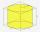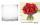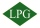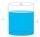# Direct relationship + cylinder - math problems

#### Number of problems found: 7

• Cylinder - h2Cylinder volume is 2.6 liters. Base area is 1.3 dm2. Calculate the height of the cylinder.
• Cylinder melted into cuboidA circular cylinder has area of cross section 56cm2 and the height is 10cm the cylinder is melted and made into a cuboid of base area 16cm2. What is the height of the cuboid?
• Cylinder-shaped vaseIf the cylinder-shaped vase is filled with water up to 35 cm, it contains 1 liter of water. How much water will it contain if it is filled to a height of 45cm?
• Liters od milkThe cylinder-shaped container contains 80 liters of milk. Milk level is 45 cm. How much milk will in the container, if level raise to height 72 cm?
• Cook on gasThe gas cylinder will last for 30 weekends for 2 hours of daily cooking. How many days will we be able to cook on a new cylinder when we cook 3 hours a day?
• CylinderThe cylinder-shaped container has 80 liters of water. The water reaches 45 cm height. How many liters of water will be in a container if water level extend to a height 72 cm? Write the result in liters, write down only as a whole or decimal number.
• PipesThe water pipe has a cross-section 1087 cm2. An hour has passed 960 m3 of water. How much water flows through the pipe with cross-section 300 cm2 per 9 hours if water flows the same speed?

We apologize, but in this category are not a lot of examples.
Do you have an interesting mathematical word problem that you can't solve it? Submit a math problem, and we can try to solve it.

We will send a solution to your e-mail address. Solved examples are also published here. Please enter the e-mail correctly and check whether you don't have a full mailbox.

Please do not submit problems from current active competitions such as Mathematical Olympiad, correspondence seminars etc...

Direct relationship - math word problems. Cylinder Problems.